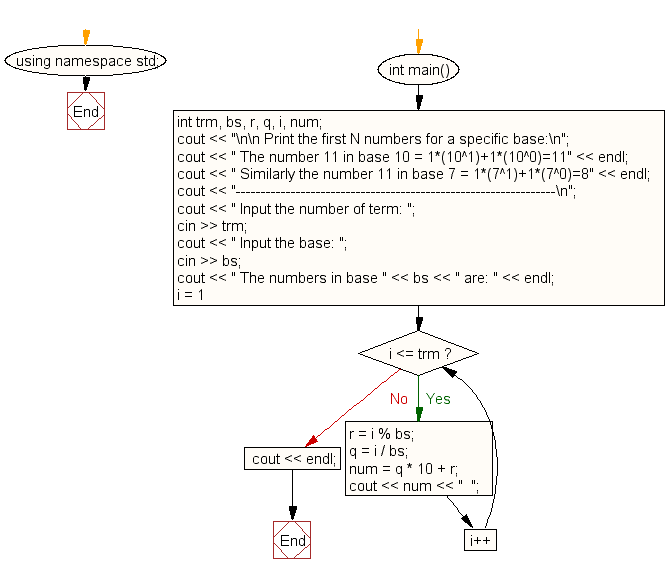﻿ C++ Exercises: Print the first N numbers for a specific base - w3resource

# C++ Exercises: Print the first N numbers for a specific base

## C++ For Loop: Exercise-68 with Solution

Write a program that will print the first N numbers for a specific base.

Sample Solution:-

C++ Code :

``````#include <iostream>
using namespace std;
int main()
{
int trm, bs, r, q, i, num;
cout << "\n\n Print the first N numbers for a specific base:\n";
cout << " The number 11 in base 10 = 1*(10^1)+1*(10^0)=11" << endl;
cout << " Similarly the number 11 in base 7 = 1*(7^1)+1*(7^0)=8" << endl;
cout << "----------------------------------------------------------------\n";
cout << " Input the number of term: ";
cin >> trm;
cout << " Input the base: ";
cin >> bs;
cout << " The numbers in base " << bs << " are: " << endl;
for (i = 1; i <= trm; i++)
{
r = i % bs;
q = i / bs;
num = q * 10 + r;
cout << num << "  ";
}
cout << endl;
}
``````

Sample Output:

``` Print the first N numbers for a specific base:
The number 11 in base 10 = 1*(10^1)+1*(10^0)=11
Similarly the number 11 in base 7 = 1*(7^1)+1*(7^0)=8
----------------------------------------------------------------
Input the number of term: 15
Input the base: 9
The numbers in base 9 are:
1  2  3  4  5  6  7  8  10  11  12  13  14  15  16
```

Flowchart:C++ Code Editor: